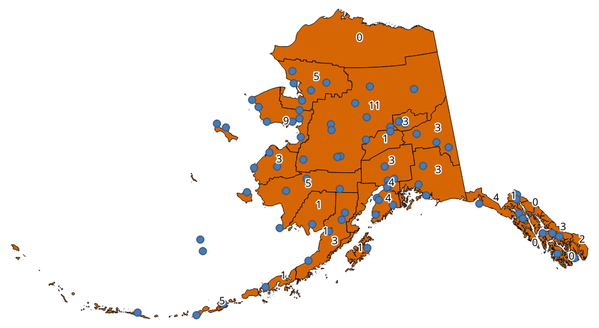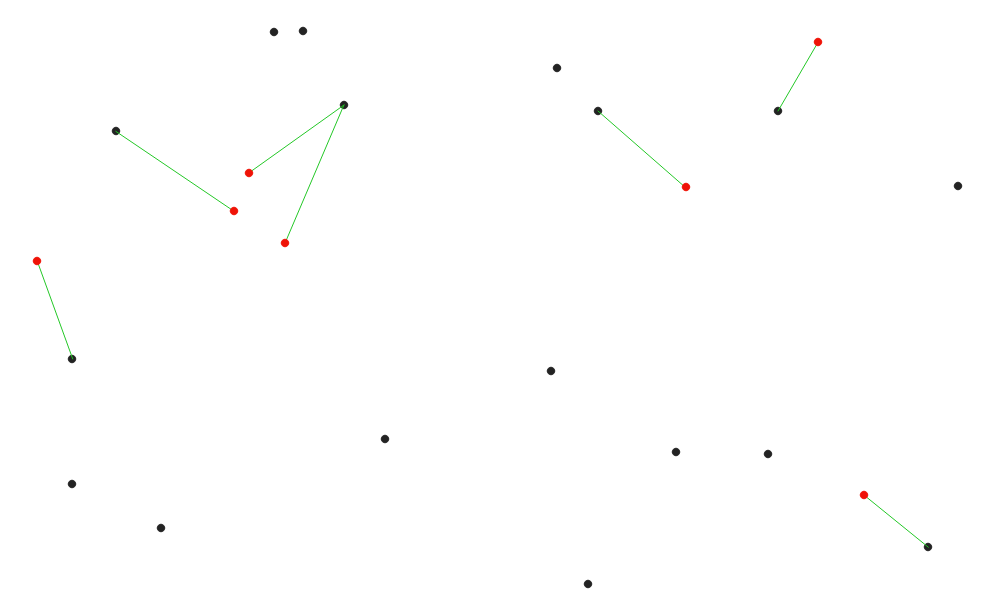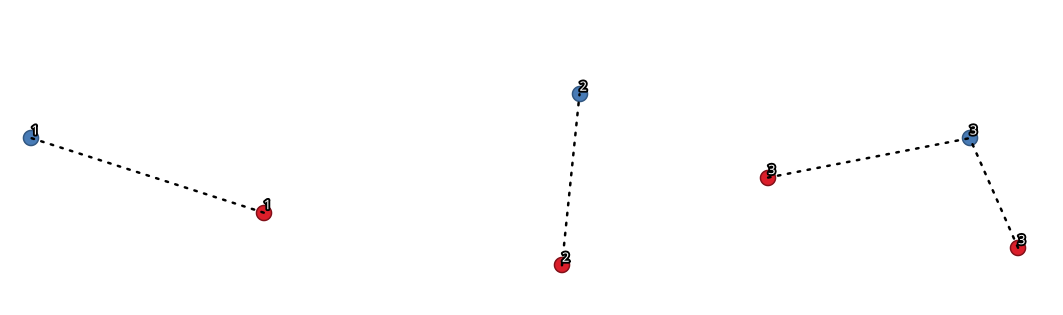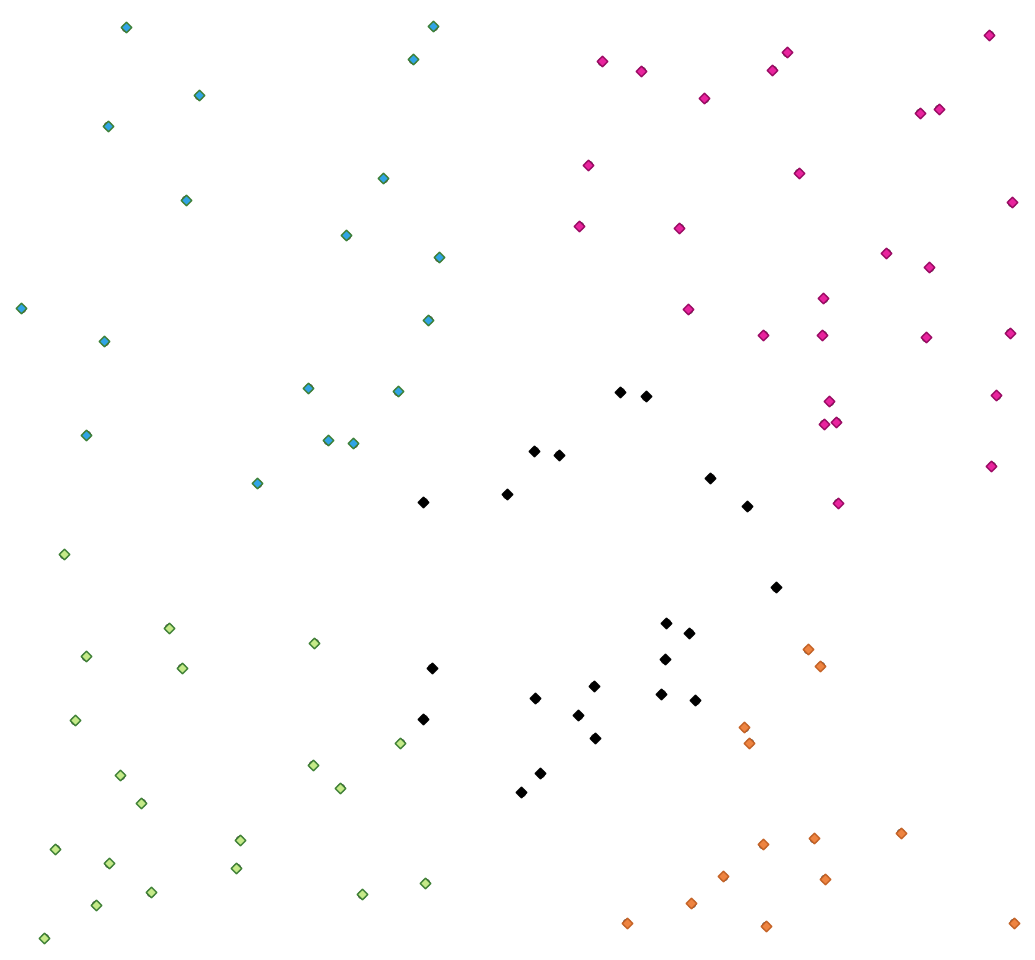Docs in progress for ‘QGIS testing’. Visit https://docs.qgis.org/3.4 for QGIS 3.4 docs and translations.

# Vector analysis¶

## Basic statistics for fields¶

Generates basic statistics for a field of the attribute table of a vector layer.

Numeric, date, time and string fields are supported.

The statistics returned will depend on the field type.

Statistics are generated as an HTML file and are available in the Processing ‣ Results viewer.

`Default menu`: Vector ‣ Analysis Tools

### Parameters¶

`Input vector` [vector: any]
Vector layer to calculate the statistic on.
`Field to calculate statistics on` [tablefield: any]
Any supported table field to calculate the statistics.

### Outputs¶

`Statistics` [html]
HTML file with calculated statistics.

## Climb along line¶

This algorithm calculates the total climb and descent along line geometries. The input layer must have Z values present. If Z values are not available, the Drape (set Z value from raster) algorithm may be used to add Z values from a DEM layer.

The output layer is a copy of the input layer with additional fields that contain the total climb (`climb`), total descent (`descent`), the minimum elevation (`minelev`) and the maximum elevation (`maxelev`) for each line geometry. If the input layer contains fields with the same names as these added fields, they will be renamed (field names will be altered to “name_2”, “name_3”, etc, finding the first non-duplicate name).

### Parameters¶

`Line layer` [vector: line]
Line layer to calculate the climb for. Must have Z values.

### Outputs¶

`Climb layer` [vector: line]
Line layer containing new attributes with the results from climb calculations. Key in the result dictionary: `OUTPUT`.
`Total climb` [number]
The sum of the climb for all the line geometries in the layer. Key in the result dictionary: `TOTALCLIMB`.
`Total descent` [number]
The sum of the descent for all the line geometries in the layer. Key in the result dictionary: `TOTALDESCENT`.
`Minimum elevation` [number]
The minimum elevation for the geometries in the layer. Key in the result dictionary: `MINELEVATION`.
`Maximum elevation` [number]
The maximum elevation for the geometries in the layer. Key in the result dictionary: `MAXELEVATION`.

## Count points in polygon¶

Takes a point and a polygon layer and counts the number of points from the first one in each polygon of the second one.

A new polygons layer is generated, with the exact same content as the input polygons layer, but containing an additional field with the points count corresponding to each polygon.The labels identify the point count

An optional weight field can be used to assign weights to each point. Alternatively, a unique class field can be specified. If both options are used, the weight field will take precedence and the unique class field will be ignored.

`Default menu`: Vector ‣ Analysis Tools

### Parameters¶

`Polygons` [vector: polygon]
Polygon layer whose features are associated with the count of points they contain.
`Points` [vector: point]
Point layer to count features from.
`Weight field` [tablefield: any]

Optional

A field with numeric-like values from the point layer. The count generated will be the sum of the weight field for each point contained by the polygon.

`Class field` [tablefield: any]

Optional

Points are classified based on the selected attribute and if several points with the same attribute value are within the polygon, only one of them is counted. The final count of the points in a polygon is, therefore, the count of different classes that are found in it.

`Count field name` [string]

The name of the field to store the count of points.

Default: NUMPOINTS

### Outputs¶

`Count` [vector: polygon]
Resulting layer with the attribute table containing the new column of the points count.

## DBSCAN clustering¶

Clusters point features based on a 2D implementation of Density-based spatial clustering of applications with noise (DBSCAN) algorithm.

The algorithm requires two parameters, a minimum cluster size, and the maximum distance allowed between clustered points.

### Parameters¶

`Input layer` [vector: point]
Layer to analyze.
`Minimum cluster size` [number]

Minimum number of features to generate a cluster.

Default: 5

`Maximum distance between clustered points` [number]

Distance beyond which two features can not belong to the same cluster.

Default: 1.0

`Treat border points as noise (DBSCAN*)` [boolean]

Optional

If checked, points on the border of a cluster are themselves treated as unclustered points, and only points on the interior of a cluster are tagged as clustered.

Default: FALSE

`Cluster field name` [string]

Name of the field where to store the associated cluster number.

Default: CLUSTER_ID

### Outputs¶

`Clusters` [vector: point]
Vector layer containing the original features with a field setting the cluster they belong to.

K-means clustering

## Distance matrix¶

Calculates for point features distances to their nearest features in the same layer or in another layer.

`Default menu`: Vector ‣ Analysis Tools

### Parameters¶

`Input point layer` [vector: point]
Point layer for which the distance matrix is calculated (from points).
`Input unique ID field` [tablefield: any]
Field to use to uniquely identify features of the input layer. Used in the output attribute table.
`Target point layer` [vector: point]
Point layer containing the nearest point(s) to search (to points).
`Target unique ID field` [tablefield: any]
Field to use to uniquely identify features of the target layer. Used in the output attribute table.
`Output matrix type` [enumeration]

Different types of calculation are available:

• 0 — Linear (N * k x 3) distance matrix: for each input point, reports the distance to each of the k nearest target points. The output matrix consists of up to k rows per input point, and each row has three columns: InputID, TargetID and Distance.
• 1 — Standard (N x T) distance matrix
• 2 — Summary distance matrix (mean, std. dev., min, max): for each input point, reports statistics on the distances to its target points.

Default: 0

`Use only the nearest (k) target points` [number]

You can choose to calculate the distance to all the points in the target layer or limit to a number (k) of closest features.

Default: 0 — all the points are used.

### Outputs¶

`Distance matrix` [vector: point]
Point (or MultiPoint for the “Linear (N * k x 3)” case) vector layer containing the distance calculation for each input feature. Its features and attribute table depend on the selected output matrix type.

## Distance to nearest hub (line to hub)¶

Creates lines that join each feature of an input vector to the nearest feature in a destination layer. Distances are calculated based on the center of each feature.Display the nearest hub for the red input features

### Parameters¶

`Source points layer` [vector: any]
Vector layer for which the nearest feature is searched.
`Destination hubs layer` [vector: any]
Vector layer containing the features to search for.
`Hub layer name attribute` [tablefield: any]
Field to use to uniquely identify features of the destination layer. Used in the output attribute table.
`Measurement unit` [enumeration]

Units in which to report the distance to the closest feature:

• 0 — Meters
• 1 — Feet
• 2 — Miles
• 3 — Kilometers
• 4 — Layer units

Default: 0

### Outputs¶

`Hub distance` [vector: line]
Line vector layer with the attributes of the input features, the identifier of their closest feature and the calculated distance.

## Distance to nearest hub (points)¶

Creates a point layer representing the center of the input features with the addition of two fields containing the identifier of the nearest feature (based on its center point) and the distance between the points.

### Parameters¶

`Source points layer` [vector: any]
Vector layer for which the nearest feature is searched.
`Destination hubs layer` [vector: any]
Vector layer containing the features to search for.
`Hub layer name attribute` [tablefield: any]
Field to use to uniquely identify features of the destination layer. Used in the output attribute table.
`Measurement unit` [enumeration]

Units in which to report the distance to the closest feature:

• 0 — Meters
• 1 — Feet
• 2 — Miles
• 3 — Kilometers
• 4 — Layer units

Default: 0

### Outputs¶

`Hub distance` [vector: point]
Point vector layer with the attributes of the input features, the identifier of their closest feature and the calculated distance.

## Join by lines (hub lines)¶

Creates hub and spoke diagrams by connecting lines from points on the spoke layer to matching points in the hub layer.

Determination of which hub goes with each point is based on a match between the Hub ID field on the hub points and the Spoke ID field on the spoke points.

If input layers are not point layers, a point on the surface of the geometries will be taken as the connecting location.Join points on common field

### Parameters¶

`Hub point layer` [vector: any]
Input layer.
`Hub ID field` [tablefield: any]
Field of the hub layer with ID to join.
`Hub layer fields to copy`

Optional

Choose here the field(s) of the hub layer to copy. If no field(s) are chosen all fields are taken.

`Spoke point layer` [vector: any]
`Spoke ID field` [tablefield: any]
Field of the spoke layer with ID to join.
`Spoke layer fields to copy`

Optional

Field(s) of the spoke layer to be copied. If no fields are chosen all fields are taken.

### Outputs¶

`Hub lines` [vector: lines]
The resulting line layer.

## K-means clustering¶

Calculates the 2D distance based k-means cluster number for each input feature.

K-means clustering aims to partition the features into k clusters in which each feature belongs to the cluster with the nearest mean. The mean point is represented by the barycenter of the clustered features.

If input geometries are lines or polygons, the clustering is based on the centroid of the feature.A five class point clusters

### Parameters¶

`Input layer` [vector: any]
Layer to analyze.
`Number of clusters` [number]

Number of clusters to create with the features.

Default: 5

`Cluster field name` [tablefield: any]

Name of the field where to store the associated cluster number.

Default: CLUSTER_ID

### Outputs¶

`Clusters` [vector: any]
Vector layer containing the original features with a field setting the cluster they belong to.

DBSCAN clustering

## List unique values¶

Lists unique values of an attribute table field and counts their number.

`Default menu`: Vector ‣ Analysis Tools

### Parameters¶

`Input layer` [vector: any]
Layer to analyze.
`Target field` [tablefield: any]
Field to analyze.

### Outputs¶

`Unique values` [table]
Summary table layer with unique values.
`HTML report` [html]
HTML report of unique values in the Processing ‣ Results viewer.

## Mean coordinate(s)¶

Computes a point layer with the center of mass of geometries in an input layer.

An attribute can be specified as containing weights to be applied to each feature when computing the center of mass.

If an attribute is selected in the parameter, features will be grouped according to values in this field. Instead of a single point with the center of mass of the whole layer, the output layer will contain a center of mass for the features in each category.

`Default menu`: Vector ‣ Analysis Tools

### Parameters¶

`Input layer` [vector: any]
Input vector layer.
`Weight field` [tablefield: numeric]

Optional

Field to use if you want to perform a weighted mean.

`Unique ID field` [tablefield: numeric]

Optional

Unique field on which the calculation of the mean will be made.

### Outputs¶

`Mean coordinates` [vector: point]
Resulting point(s) layer.

## Nearest neighbour analysis¶

Performs nearest neighbor analysis for a point layer.

Output is generated as an HTML file with the computed statistical values:

• Observed mean distance
• Expected mean distance
• Nearest neighbour index
• Number of points
• Z-Score

`Default menu`: Vector ‣ Analysis Tools

### Parameters¶

`Points` [vector: point]
Point vector layer to calculate the statistics on.

### Outputs¶

`Nearest neighbour` [html]
HTML file in output with the computed statistics.

## Statistics by categories¶

Calculates statistics of fields depending on a parent class.

The output is a table layer with the following statistics calculated:

• count
• unique
• min
• max
• range
• sum
• mean
• median
• stdev
• minority
• majority
• q1
• q3
• iqr

### Parameters¶

`Input vector layer` [vector: any]
Input vector layer with unique classes and values.
`Field to calculate the statistics on` [tablefield: any]

Optional

If empty only the count will be calculated.

`Field(s) with categories` [tablefield: any] [list]
Field(s) of the categories.

### Outputs¶

`N unique values` [table]
Table with statistics field.

## Sum line lengths¶

Takes a polygon layer and a line layer and measures the total length of lines and the total number of them that cross each polygon.

The resulting layer has the same features as the input polygon layer, but with two additional attributes containing the length and count of the lines across each polygon.

The names of these two fields can be configured in the algorithm parameters.

`Default menu`: Vector ‣ Analysis Tools

### Parameters¶

`Lines` [vector: line]
Input vector line layer.
`Polygons` [vector: polygon]
Polygon vector layer.
`Lines length field name` [string]

Name of the field of the lines length.

Default: LENGTH

`Lines count field name` [string]

Name of the field of the lines count.

Default: COUNT

### Outputs¶

`Line length` [vector: polygon]
Polygon output layer with fields of lines length and line count.